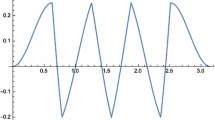# Quadratic functions fulfilling an additional condition along hyperbolas or the unit circle

## Abstract

Let $$S_1$$ denote the set of all pairs (xy) of real numbers that fulfill the condition $$x^2 - y^2 = 1$$, and $$S_2$$ denote the set of all pairs (xy) of real numbers that fulfill the condition $$x^2 + y^2 = 1 \,$$. In this paper we consider quadratic real functions f that satisfy the additional equation $$y^2 f(x) = x^2 f(y)$$ under the condition $$(x,y) \in S_i$$$$(i=1,2)$$. We prove that each of these conditions implies $$f(x) = f(1) x^2$$ for all $$x \in \mathbb {R}$$.

This is a preview of subscription content, log in via an institution to check access.

Price excludes VAT (USA)
Tax calculation will be finalised during checkout.

### Quadratic functions fulfilling an additional condition along the hyperbola $$\pmb {xy = 1}$$

Zoltán Boros & Edit Garda-Mátyás### A Note on Successive Coefficients of Convex Functions

Ming Li & Toshiyuki Sugawa

D. Yu. Karamzin

## References

1. Aczél, J.: Some unsolved problems in the theory of functional equations. Arch. Math. 15, 435–444 (1964)

2. Aczél, J.: The general solution of two functional equations by reduction to functions additive in two variables and with the aid of Hamel bases. Glasnik Mat.-Fiz. Astronom. Ser. II Društvo Mat. Fiz. Hrvatske 20, 65–73 (1965)

3. Aczél, J., Dhombres, J.: Functional equations in several variables. Encyclopaedia of Mathematics and its Applications, vol. 31. Cambridge University Press, Cambridge (1989).

4. Benz, W.: 5. Problem in report of meeting: the twenty-seventh international symposium on functional equations. Aequ. Math. 39, 302 (1990)

5. Boros, Z., Erdei, P.: A conditional equation for additive functions. Aequ. Math. 70, 309–313 (2005)

6. Boros, Z., Fechner, W.: An alternative equation for polynomial functions. Aequ. Math. 89(1), 17–22 (2015)

7. Boros, Z., Fechner, W., Kutas, P.: A regularity condition for quadratic functions involving the unit circle. Publ. Math. Debr. 89(3), 297–306 (2016)

8. Boros, Z., Garda-Mátyás, E.: Conditional equations for quadratic functions. Acta Math. Hung. 154(2), 389–401 (2018)

9. Jurkat, W.B.: On Cauchy’s functional equation. Proc. Am. Math. Soc. 16, 683–686 (1965)

10. Kominek, Z., Reich, L., Schwaiger, J.: On additive functions fulfilling some additional condition. Sitzungsber. Abt. II(207), 35–42 (1998)

11. Kuczma, M.: An Introduction to the Theory of Functional Equations and Inequalities, 2nd edn. Birkhäuser, Basel (2009)

12. Kutas, P.: Algebraic conditions for additive functions over the reals and over finite fields. Aequ. Math. 92, 563–575 (2018)

13. Kurepa, S.: The Cauchy functional equation and scalar product in vector spaces. Glasnik Mat.-Fiz. Astronom. Ser. II Društvo Mat. Fiz. Hrvatske 19, 23–36 (1964)

14. Kurepa, S.: Remarks on the Cauchy functional equation. Publ. Inst. Math. (Beograd) (N.S.) 5(19), 85–88 (1965)

15. Nishiyama, A., Horinouchi, S.: On a system of functional equations. Aequ. Math. 1, 1–5 (1968)

## Acknowledgements

The author would like to thank Zoltán Boros for helpful suggestions and his constant support and also the anonymous referee for his/her essential comments and useful advice.

## Author information

Authors

### Corresponding author

Correspondence to Edit Garda-Mátyás.

## Rights and permissions

Reprints and Permissions